xehoth 明日は明日の风が吹く 2021-02-19T08:13:47.080Z https://blog.xehoth.cc/ xehoth Hexo 法线贴图与切线空间 https://blog.xehoth.cc/CG/OpenGL/TangentSpace/ 2021-02-18T10:52:43.000Z 2021-02-19T08:13:47.080Z 为了能正确使用法线贴图，我们需要在切线空间进行光照，本文记录切线的计算以及 TBN 矩阵的推导，并完善一些细节。最后分别在切线空间、世界空间、观察空间实现 ADS 光照（都实现一遍便于对各个空间有更清晰更深入的理解，并区分其优劣）。

## 法线贴图

$$R = \frac{N_x + 1}{2}, G = \frac{N_y + 1}{2}, B = \frac{N_z + 1}{2}$$

## 切线空间

### 计算切线$$\vec{e_1} = (u_1 - u_0)T + (v_1 - v_0)B\\ \vec{e_2} = (u_2 - u_0)T + (v_2 - v_0)B$$

$$\begin{bmatrix}e_{1_x} & e_{1_y} & e_{1_z} \\ e_{2_x} & e_{2_y} & e_{2_z} \end{bmatrix} = \begin{bmatrix}\Delta U_1 & \Delta V_1 \\ \Delta U_2 & \Delta V_2 \end{bmatrix}\begin{bmatrix}T_x & T_y & T_z \\ B_x & B_y & B_z\end{bmatrix}$$

\begin{aligned}\begin{bmatrix}T_x & T_y & T_z \\ B_x & B_y & B_z\end{bmatrix} &= \begin{bmatrix}\Delta U_1 & \Delta V_1 \\ \Delta U_2 & \Delta V_2 \end{bmatrix} ^ {-1} \begin{bmatrix}e_{1_x} & e_{1_y} & e_{1_z} \\ e_{2_x} & e_{2_y} & e_{2_z} \end{bmatrix}\\& = \frac{1}{\Delta U_1 \Delta V_2 - \Delta U_2 \Delta V_1}\begin{bmatrix}\Delta V_2 & -\Delta V_1 \\ -\Delta U_2 & \Delta U_1\end{bmatrix}\begin{bmatrix}e_{1_x} & e_{1_y} & e_{1_z} \\ e_{2_x} & e_{2_y} & e_{2_z} \end{bmatrix}\end{aligned}

### TBN 矩阵

$$M = \begin{bmatrix}T_x & B_x & N_x \\ T_y & B_y & N_y \\ T_z & B_z & N_z\end{bmatrix}$$

$$M^{-1} = M ^ T = \begin{bmatrix}T_x & T_y & T_z \\ B_x & B_y & B_z \\ N_x & N_y & N_z \end{bmatrix}$$

# 使用法线贴图

• 直观简单的办法：将法线从切线空间变换到世界空间，然后在世界空间中进行光照
• 更方便光照的办法：将法线从切线空间变换到观察空间，然后在观察空间中实现光照，由于在观察空间中摄像机总是在远点看向 $-z$，这种方法比较便于实现光照
• 通常性能更高的办法：由于光源的数量一般较少，我们可以将世界空间中的光源、摄像机变换到切线空间，然后在切线空间中实现光照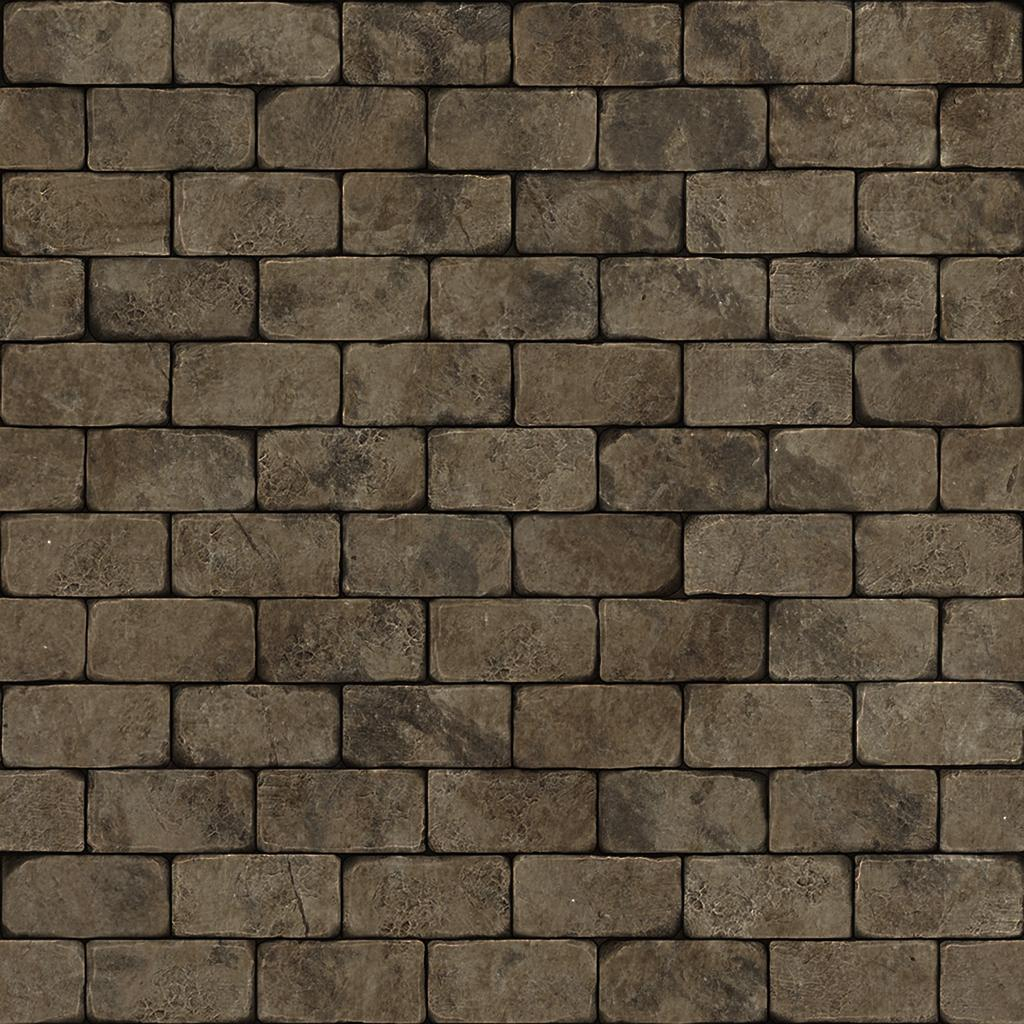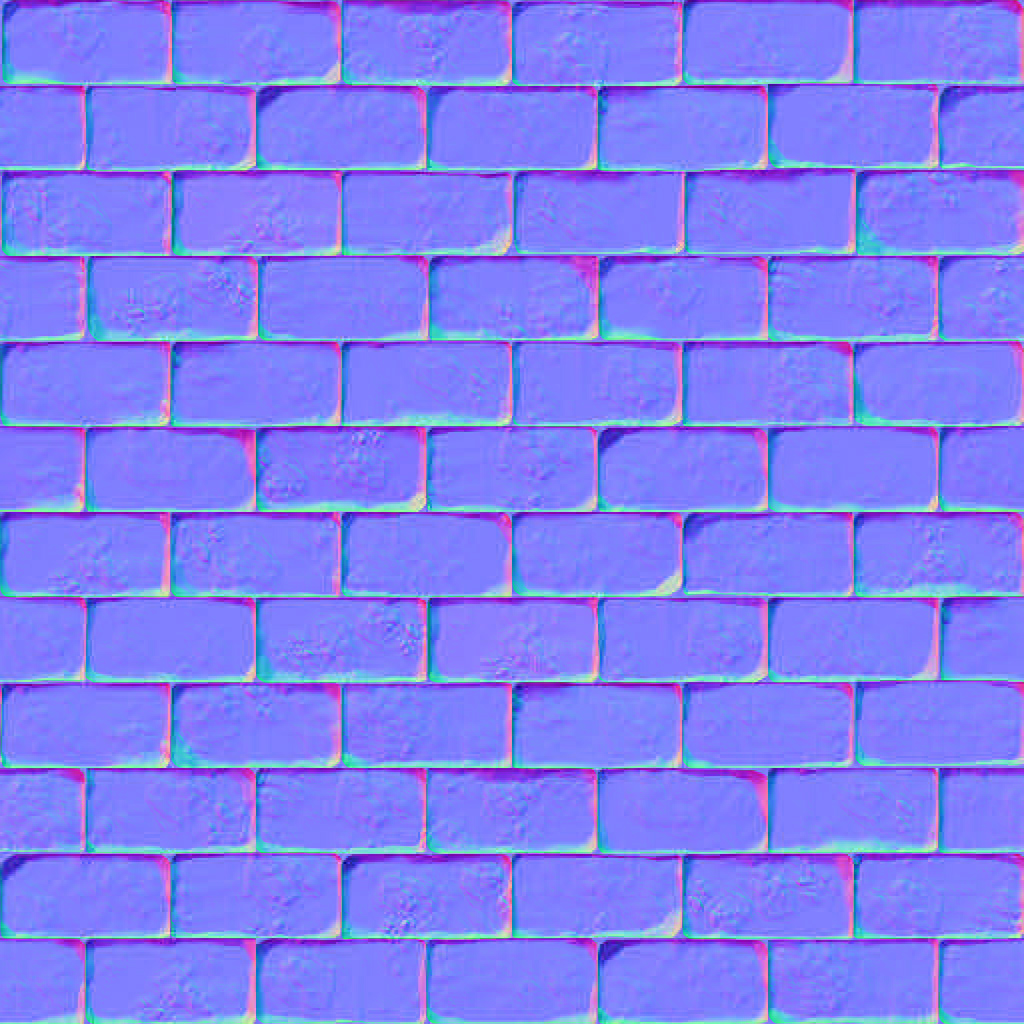## 切线空间 $\rightarrow$ 世界空间

$$T’ = T - (T \cdot N)N$$

## 参考

]]>
Transformation in OpenGL https://blog.xehoth.cc/CG/OpenGL/Transformation-OpenGL/ 2021-02-15T14:35:16.000Z 2021-02-16T06:07:14.043Z 实际在 OpenGL 中的变换的一些记录，尤其是与 「GAMES101」Transformation 推导中的一些区别。其中旋转、平移等常见变换以及视图变换均是相同的。这里主要讨论正交投影和透视投影，以及法线变换矩阵的推导。

## NDC 坐标 (Normalized Device Coordinates, 标准化设备坐标)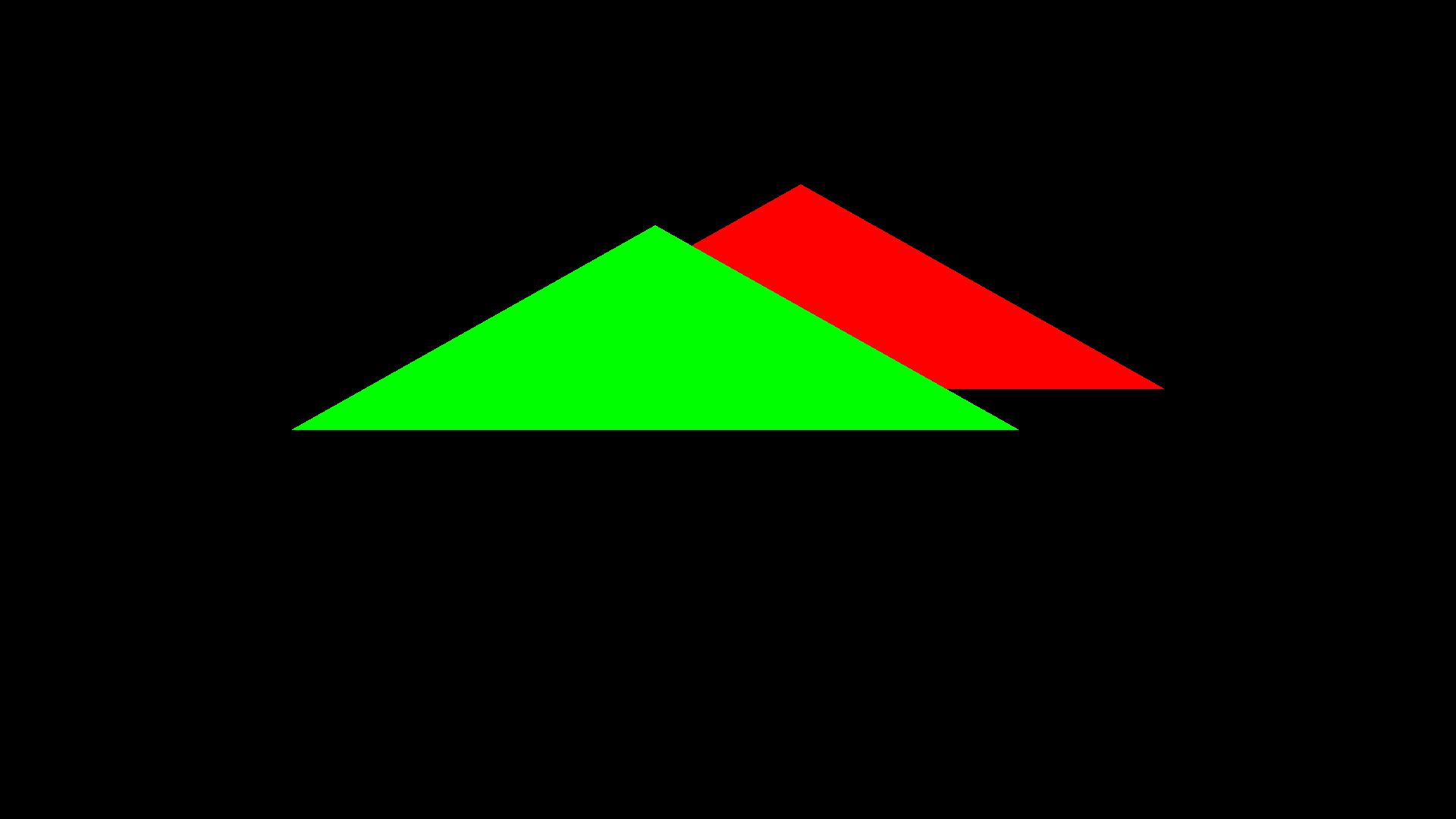## Orthographic projection (正交投影)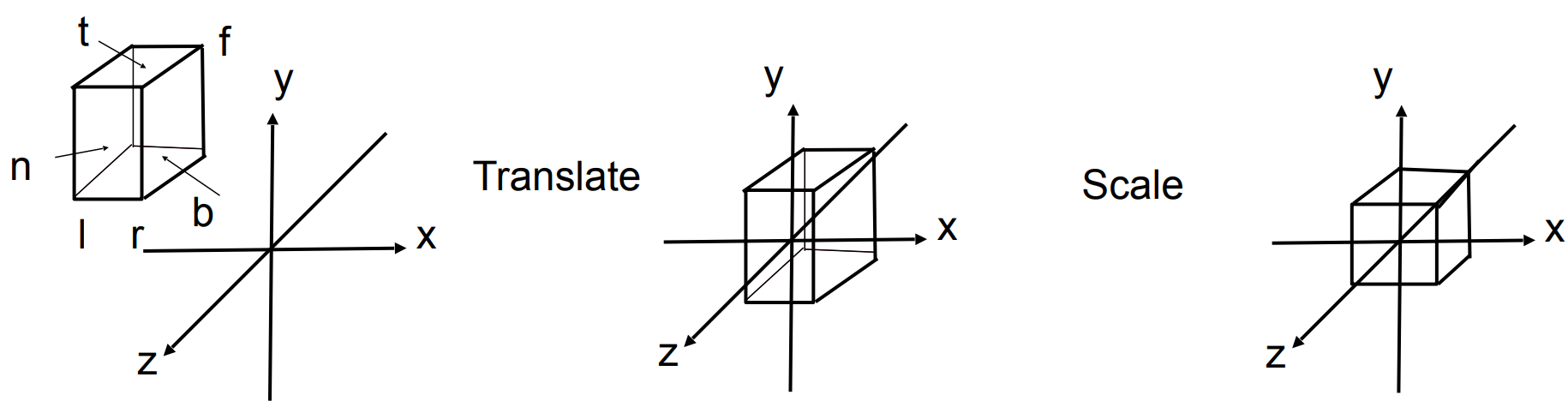\begin{aligned}M_{ortho} &= \begin{bmatrix} \frac{2}{r - l} & 0 & 0 & 0\\ 0 & \frac{2}{t - b} & 0 & 0\\ 0 & 0 & \color{red}{-\frac{2}{f - n}} & 0\\ 0 & 0 & 0 & 1\end{bmatrix}\begin{bmatrix} 1 & 0 & 0 & -\frac{r + l}{2}\\ 0 & 1 & 0 & -\frac{t + b}{2}\\ 0 & 0 & 1 & -\frac{n + f}{2}\\ 0 & 0 & 0 & 1\end{bmatrix}\\&= \begin{bmatrix}\frac{2}{r - l} & 0 & 0 & -\frac{r + l}{r - l}\\0 & \frac{2}{t - b} & 0 & -\frac{t + b}{t - b}\\0 & 0 & -\frac{2}{f - n} & -\frac{f + n}{f - n}\\0 & 0 & 0 & 1\end{bmatrix}\end{aligned}

## Perspective projection (透视投影)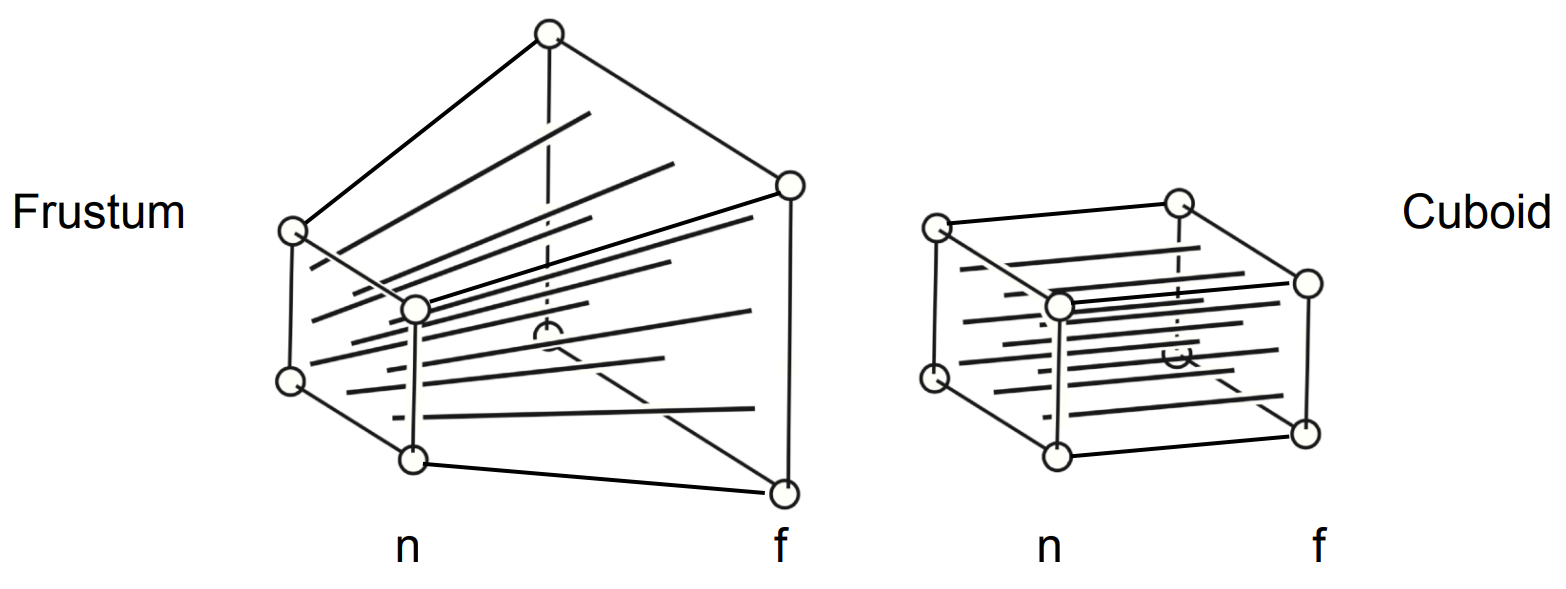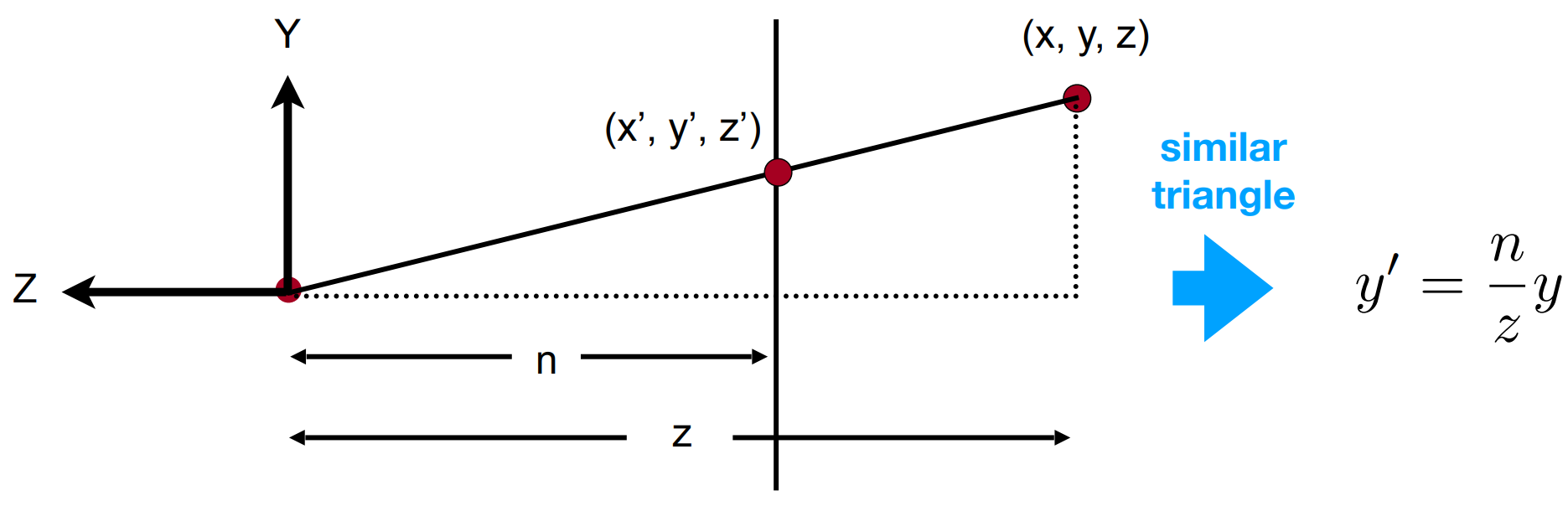$$y’ = \color{red}{\left|\frac{n}{z}\right|}y$$

$$x’ = \color{red}{\left|\frac{n}{z}\right|}x$$

$$\begin{bmatrix} x\\y\\z\\1\end{bmatrix} \Rightarrow \begin{bmatrix} \color{red}{-nx/z}\\\color{red}{-ny/z}\\\text{unknown}\\1\end{bmatrix} = ^ {\times \color{red}{-z}}\begin{bmatrix} nx\\ny\\\text{still unknown}\\\color{red}{-z}\end{bmatrix}$$

$$M_{persp \rightarrow ortho} \begin{bmatrix} x\\y\\z\\1\end{bmatrix}=\begin{bmatrix} nx\\ny\\\text{unknown}\\z\end{bmatrix}$$

$$M_{persp \rightarrow ortho}=\begin{bmatrix} n & 0 & 0 & 0\\ 0 & n & 0 & 0\\ ? & ? & ? & ?\\ 0 & 0 & \color{red}{-1} & 0\end{bmatrix}$$

$$\begin{bmatrix} x\\y\\-n\\1\end{bmatrix} \Rightarrow \begin{bmatrix} x\\y\\-n\\1\end{bmatrix} = ^ {\times (z=n)}\begin{bmatrix} nx\\ny\\-n^2\\n\end{bmatrix}$$

$$\begin{bmatrix} 0 & 0 & A & B\end{bmatrix}\begin{bmatrix} x\\y\\-n\\1\end{bmatrix}=-n^2$$

$$-An + B = -n ^ 2$$

$$\begin{bmatrix} 0\\0\\-f\\1\end{bmatrix} \Rightarrow \begin{bmatrix} 0\\0\\-f\\1\end{bmatrix} = \begin{bmatrix} 0\\0\\-f^2\\f\end{bmatrix}$$

$$\begin{bmatrix} 0 & 0 & A & B\end{bmatrix}\begin{bmatrix} 0\\0\\-f\\1\end{bmatrix}=-f^2$$

$$-Af + B = -f ^ 2$$

$$\begin{cases} -An + B = -n ^ 2\\ -Af + B = -f ^ 2\end{cases}$$

$$A = n + f, B = nf$$

$$M_{persp \rightarrow ortho}=\begin{bmatrix} n & 0 & 0 & 0\\ 0 & n & 0 & 0\\ 0 & 0 & n + f & nf\\ 0 & 0 & -1 & 0\end{bmatrix}$$

$$M_{persp} = M_{ortho}M_{persp \rightarrow ortho} = \begin{bmatrix}\frac{2n}{r - l} & 0 & \frac{r + l}{r - l} & 0\\0 & \frac{2n}{t - b} & \frac{t + b}{t - b} & 0\\0 & 0 & -\frac{f + n}{f - n} & -\frac{2nf}{f - n}\\0 & 0 & -1 & 0\end{bmatrix}$$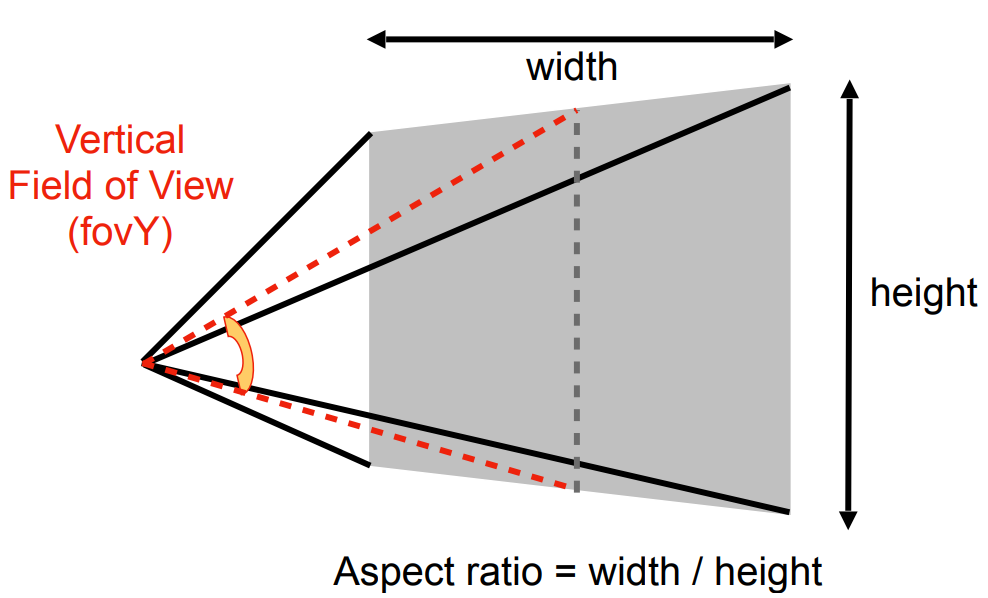• aspect ratio 宽高比
• field-of-view fov 垂直可视角度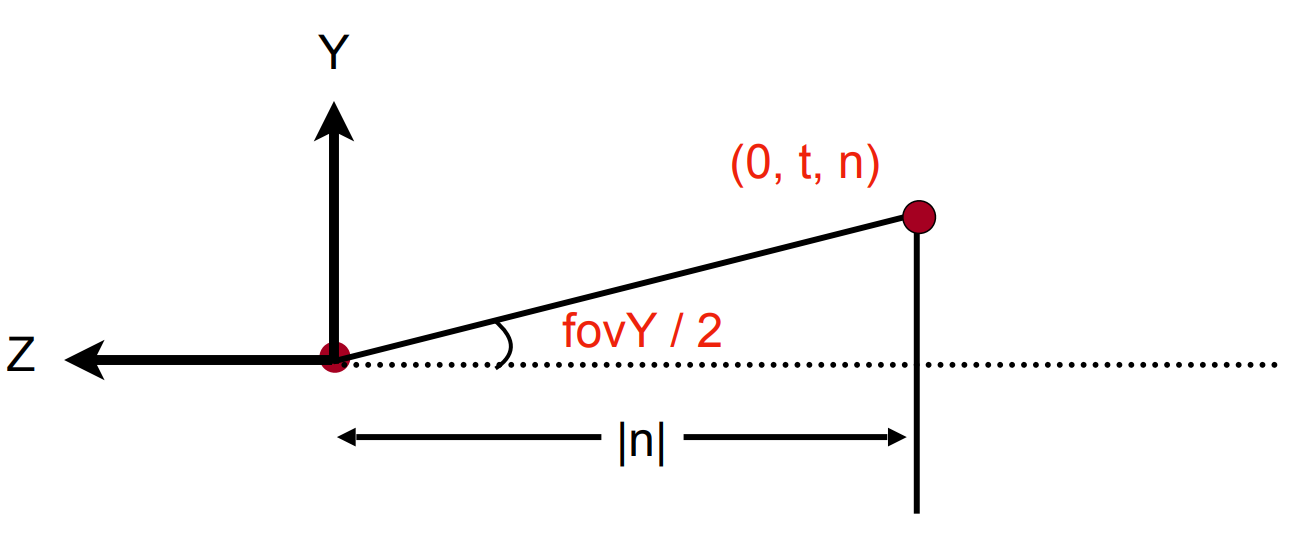$$\tan \frac{fovY}{2} = \frac{t}{\left|n\right|}$$$$aspect = \frac{r}{t}$$

$$M_{persp} = \begin{bmatrix}\frac{1}{\tan(\text{fov} / 2)\cdot \text{aspect}} & 0 & 0 & 0\\0 & \frac{1}{\tan(\text{fov} / 2)} & 0 & 0 \\0 & 0 & - \frac{f + n}{f - n} & -\frac{2fn}{f - n}\\0 & 0 & -1 & 0\end{bmatrix}$$

## 法线变换矩阵 (The Normal Transformation Matrix)

$$\boldsymbol{N’} \cdot \boldsymbol{T’} = (G \boldsymbol{N}) \cdot (M \boldsymbol{T}) = (G\boldsymbol{N})^T(M \boldsymbol{T}) = \boldsymbol N ^ T G^T M \boldsymbol T = \boldsymbol N \cdot \boldsymbol T = 0$$

$$G^TM = I \Rightarrow G = (M^{-1})^T$$

]]>
<p>实际在 OpenGL 中的变换的一些记录，尤其是与 <a href="https://blog.xehoth.cc/CG/GAMES101/Note-Transformation/">「GAMES101」Transformation</a> 推导中的一些区别。其中旋转、平移等常见变换以及视图变换均是相同的。这里主要讨论正交投影和透视投影，以及法线变换矩阵的推导。</p>
「GAMES101」Transformation https://blog.xehoth.cc/CG/GAMES101/Note-Transformation/ 2020-03-03T10:32:07.000Z 2021-02-16T04:43:03.255Z 关于变换的一些笔记。

## 2D Transformation

### Scale

$$\begin{bmatrix} y'\\x'\end{bmatrix} =\begin{bmatrix} s_x & 0\\ 0 & s_y\end{bmatrix}\begin{bmatrix} x\\y\end{bmatrix}$$

### Reflection

$$\begin{bmatrix} x'\\y'\end{bmatrix} =\begin{bmatrix} -1 & 0\\ 0 & 1\end{bmatrix}\begin{bmatrix} x\\y\end{bmatrix}$$

### Shear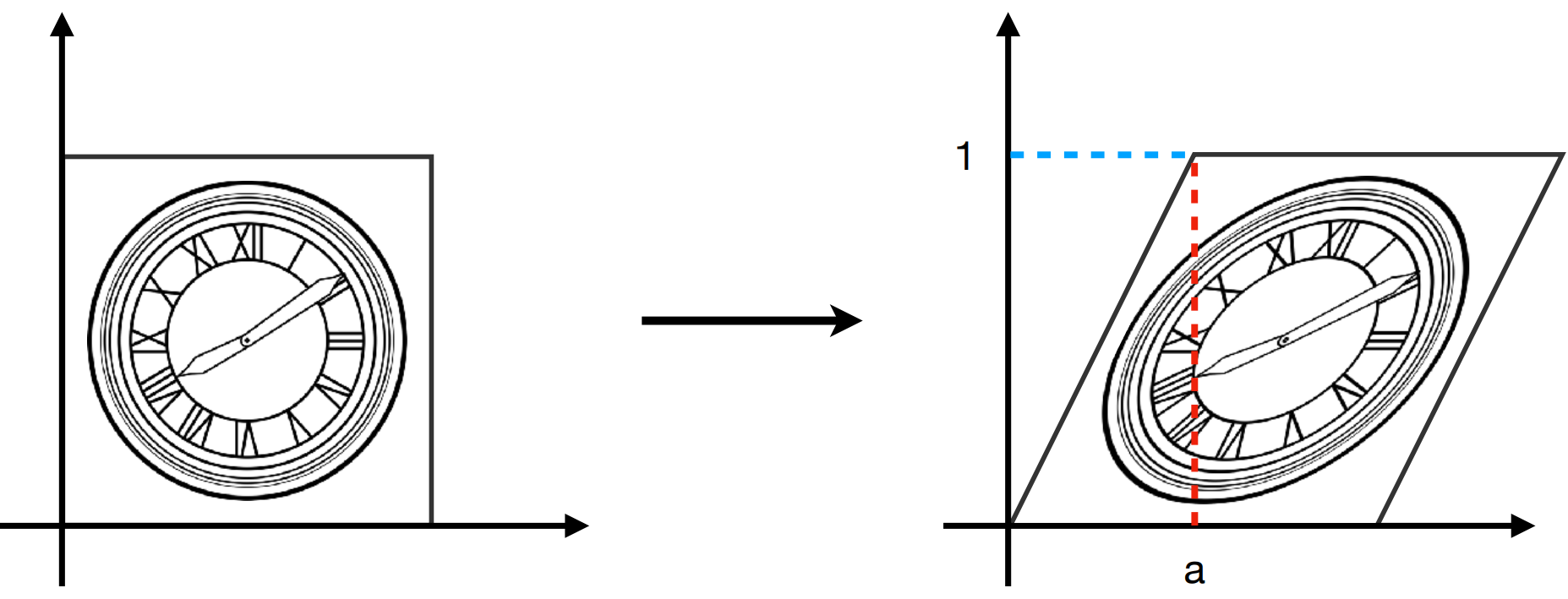$$\begin{bmatrix} x'\\y'\end{bmatrix} =\begin{bmatrix} 1 & a\\ 0 & 1\end{bmatrix}\begin{bmatrix} x\\y\end{bmatrix}$$

### Rotate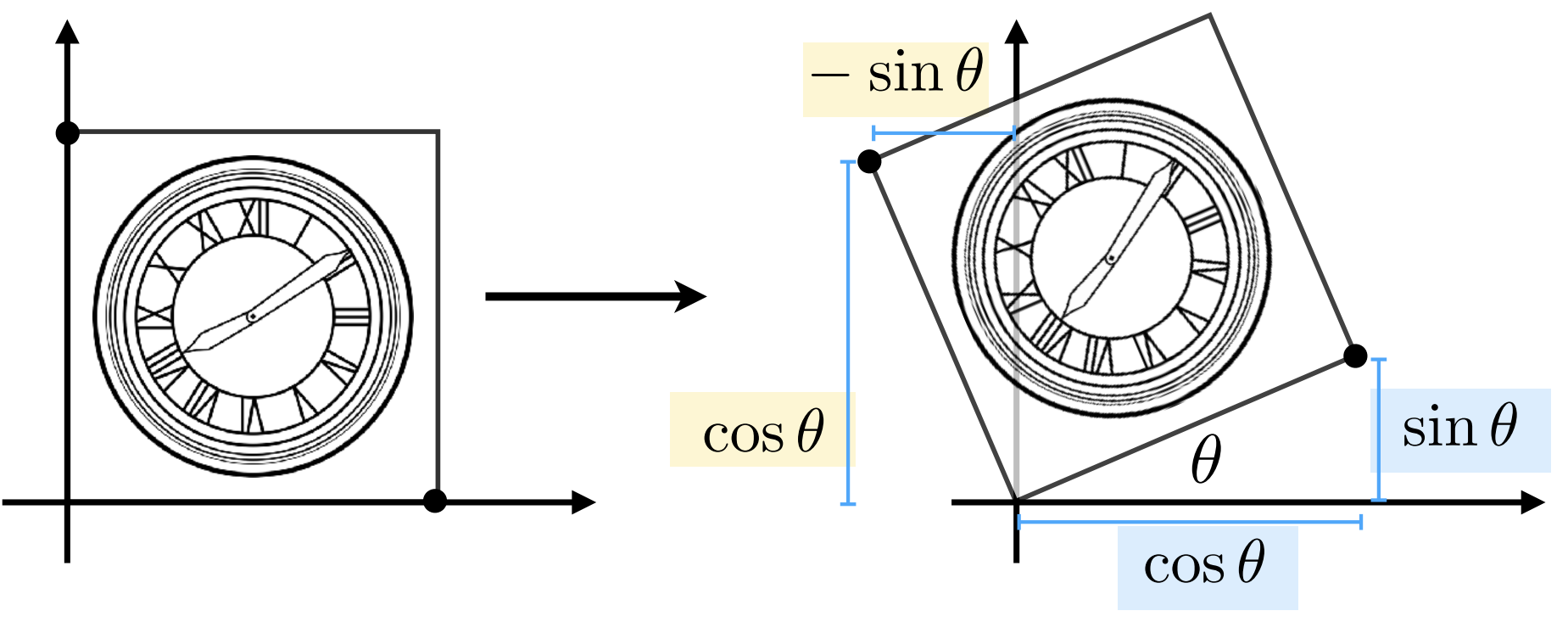$$\begin{bmatrix} x'\\y'\end{bmatrix} =\begin{bmatrix} \cos \theta & -\sin \theta\\ \sin \theta & \cos \theta\end{bmatrix}\begin{bmatrix} x\\y\end{bmatrix}$$

：$R ^ {-1}(\theta) = R(-\theta) = R ^ T(\theta)$

## Homogeneous Coordinates

### Why

$$\begin{bmatrix} x'\\y'\end{bmatrix} =\begin{bmatrix} a & b\\ c & d\end{bmatrix}\begin{bmatrix} x\\y\end{bmatrix} +\begin{bmatrix} t_x\\t_y\end{bmatrix}$$

• 2D Point = $(x, y, 1) ^ T$
• 2D vector = $(x, y, 0) ^ T$

$$\begin{bmatrix} x'\\y'\\w'\end{bmatrix} =\begin{bmatrix} 1 & 0 & t_x\\ 0 & 1 & t_y\\ 0 & 0 & 1\end{bmatrix}\begin{bmatrix} x\\y\\1\end{bmatrix} =\begin{bmatrix} x + t_x\\ y + t_y\\ 1\end{bmatrix}$$

### Affine Transformations

Affine map = linear map + translation

$$\begin{bmatrix} x'\\y'\end{bmatrix} =\begin{bmatrix} a & b\\ c & d\end{bmatrix}\begin{bmatrix} x\\y\end{bmatrix} +\begin{bmatrix} t_x\\t_y\end{bmatrix}$$

Using homogeneous coordinates:

$$\begin{bmatrix} x'\\y'\\1\end{bmatrix} =\begin{bmatrix} a & b & t_x\\ c & d & t_y\\ 0 & 0 & 1\end{bmatrix}\begin{bmatrix} x\\y\\1\end{bmatrix}$$

### 一些规律

• 最后一行是 $0, 0, 1$（二维情况下的仿射变换）
• 平移是最后一列
• 左上角 $2 \times 2$ 子矩阵为原来的变换矩阵

Scale

$$S(s_x, s_y) =\begin{bmatrix} s_x & 0 & 0\\ 0 & s_y & 0\\ 0 & 0 & 1\end{bmatrix}$$

Rotation

$$R(\alpha) =\begin{bmatrix} \cos \alpha & -\sin \alpha\\ \sin \alpha & \cos \alpha\end{bmatrix}$$

Translation

$$T(t_x, t_y) =\begin{bmatrix} 1 & 0 & t_x\\ 0 & 1 & t_y\\ 0 & 0 & 1\end{bmatrix}$$

## Composing Transform

### Decomposing Complex Transforms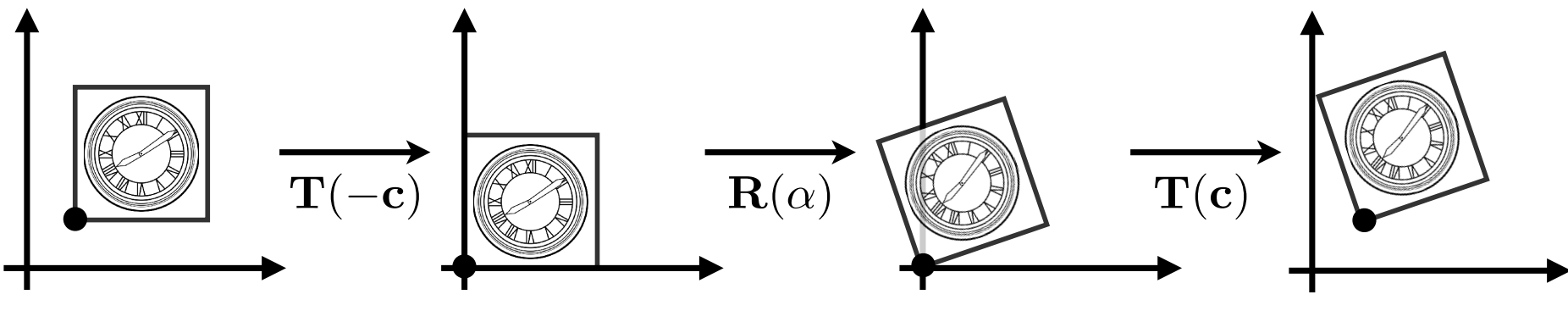$$T(c) R(\alpha) T(-c)$$

## 3D Transformation

• 3D point = $(x, y, z, 1) ^ T$
• 3D vector = $(x, y, z, 0) ^ T$

$$\begin{bmatrix} x'\\y'\\z'\\1\end{bmatrix}=\begin{bmatrix} a & b & c & t_x\\ d & e & f & t_y\\ g & h & i & t_z\\ 0 & 0 & 0 & 1\end{bmatrix}\begin{bmatrix} x\\y\\z\\1\end{bmatrix}$$

### Rotation

$$R_x(\alpha) = \begin{bmatrix} 1 & 0 & 0 & 0\\ 0 & \cos \alpha & -\sin \alpha & 0\\ 0 & \sin \alpha & \cos \alpha & 0\\ 0 & 0 & 0 & 1\end{bmatrix}$$$$R_y(\alpha) = \begin{bmatrix} \cos \alpha & 0 & \sin \alpha & 0\\ 0 & 1 & 0 & 0\\ -\sin \alpha & 0 & \cos \alpha & 0\\ 0 & 0 & 0 & 1\end{bmatrix}$$$$R_z(\alpha) = \begin{bmatrix} \cos \alpha & -\sin \alpha & 0 & 0\\ \sin \alpha & \cos \alpha & 0 & 0\\ 0 & 0 & 1 & 0\\ 0 & 0 & 0 & 1\end{bmatrix}$$

$$R_{xyz}(\alpha, \beta, \gamma) = R_x(\alpha)R_y(\beta)R_z(\gamma)$$

### Rodrigues’ Rotation Formula

$$R(\vec n, \alpha) = \cos(\alpha) \mathrm{I} + (1 - \cos \alpha)\vec n \vec n ^ T + \sin(\alpha)\begin{bmatrix} 0 & -n_z & n_y\\ n_z & 0 & -n_x\\ -n_y & n_x & 0\end{bmatrix}$$

## View / Camera transformation (视图变换)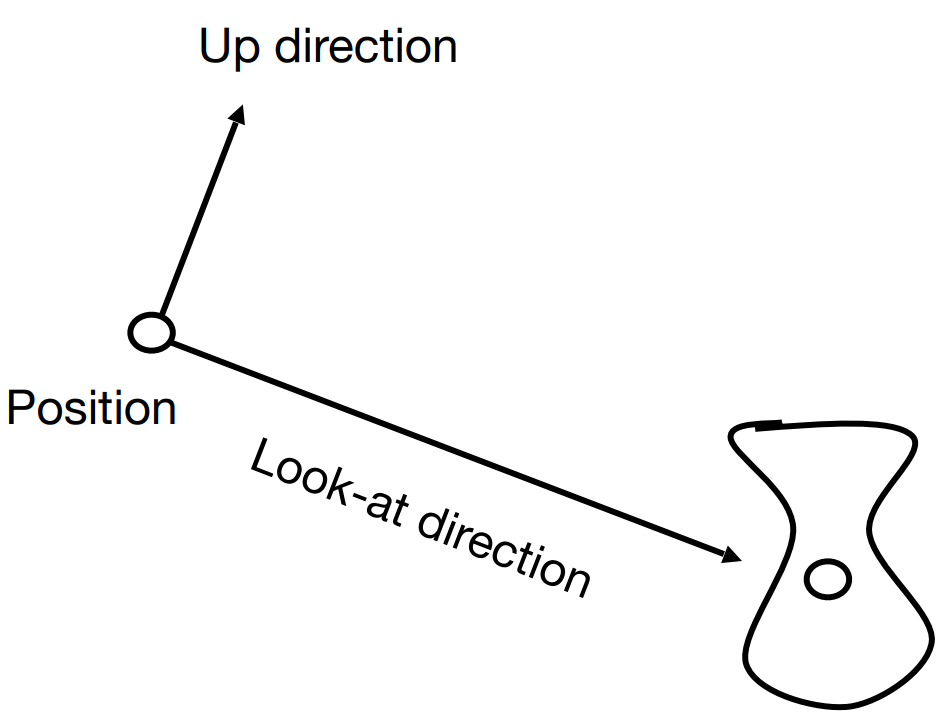• Position $\vec e$
• Look-at $\hat{g}$
• Up direction $\hat{t}$ (与 look-at 垂直)

• 平移 $\vec{e}$ 到原点
• 旋转 $\hat{g}$ 到 $-z$
• 旋转 $\hat{t}$ 到 $y$
• 旋转 $\hat{g} \times \hat{t}$ 到 $x$

$$M_{view} = R_{view}T_{view}$$

$$T_{view} = \begin{bmatrix} 1 & 0 & 0 & -x_e\\ 0 & 1 & 0 & -y_e\\ 0 & 0 & 1 & -z_e\\ 0 & 0 & 0 & 1\end{bmatrix}$$

$$R ^ {-1}_{view} = \begin{bmatrix} x_{\hat{g} \times \hat{t}} & x_{\hat{t}} & x_{-\hat{g}}& 0\\ y_{\hat{g} \times \hat{t}} & y_{\hat{t}} & y_{-\hat{g}} & 0\\ z_{\hat{g} \times \hat{t}} & z_{\hat{t}} & z_{-\hat{g}} & 0\\ 0 & 0 & 0 & 1\end{bmatrix}$$

$$R_{view} = (R ^ {-1}_{view}) ^ T = \begin{bmatrix} x_{\hat{g} \times \hat{t}} & y_{\hat{g} \times \hat{t}} & z_{\hat{g} \times \hat{t}} & 0\\ x_{\hat{t}} & y_{\hat{t}} & z_{\hat{t}} & 0\\ x_{-\hat{g}}& y_{-\hat{g}} & z_{-\hat{g}} & 0\\ 0 & 0 & 0 & 1\end{bmatrix}$$

## Projection transformation (投影变换)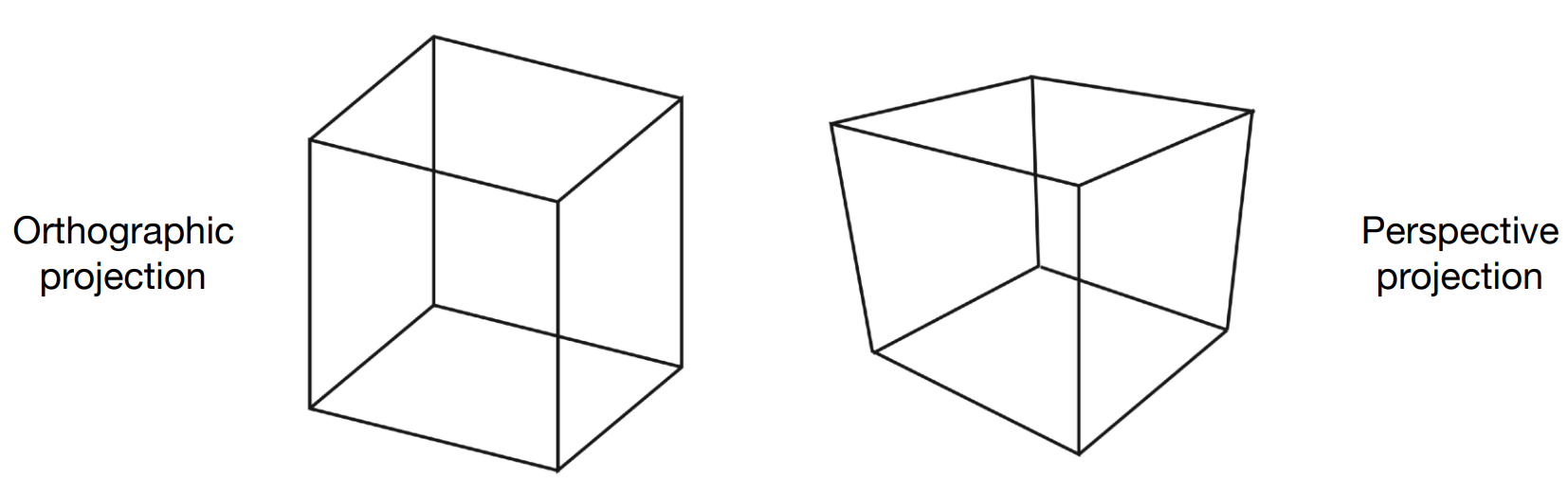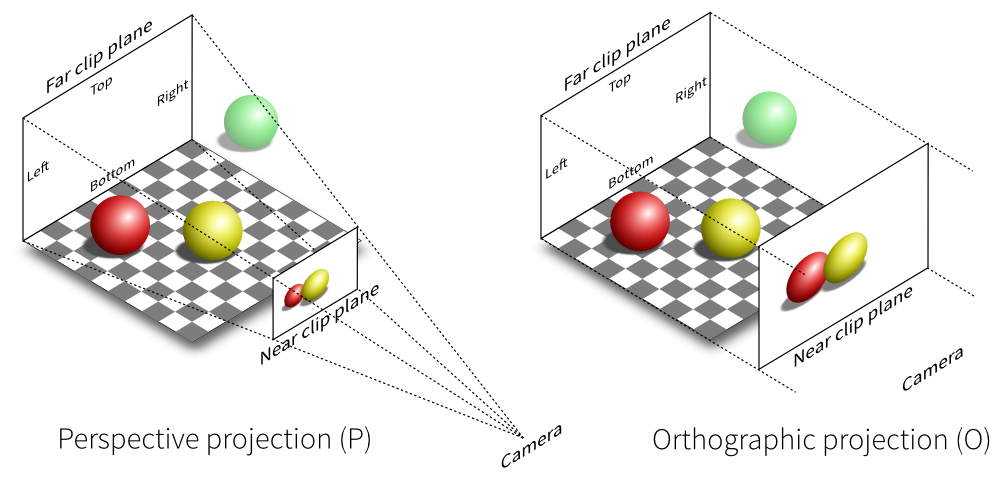### Orthographic projection (正交投影)

• 相机放在原点上，向 $-z$ 看，向上是 $y$
• 扔掉 $z$ 坐标
• 平移缩放 $x,y$ 范围至 $[-1, 1] ^ 2$$$M_{ortho} = \begin{bmatrix} \frac{2}{r - l} & 0 & 0 & 0\\ 0 & \frac{2}{t - b} & 0 & 0\\ 0 & 0 & \frac{2}{n - f} & 0\\ 0 & 0 & 0 & 1\end{bmatrix}\begin{bmatrix} 1 & 0 & 0 & -\frac{r + l}{2}\\ 0 & 1 & 0 & -\frac{t + b}{2}\\ 0 & 0 & 1 & -\frac{n + f}{2}\\ 0 & 0 & 0 & 1\end{bmatrix}$$

### Perspective projection (透视投影)$$y’ = \frac{n}{z}y$$

$$x’ = \frac{n}{z}x$$

$$\begin{bmatrix} x\\y\\z\\1\end{bmatrix} \Rightarrow \begin{bmatrix} nx/z\\ny/z\\\text{unknown}\\1\end{bmatrix} = ^ {\times z}\begin{bmatrix} nx\\ny\\\text{still unknown}\\z\end{bmatrix}$$

$$M_{persp \rightarrow ortho} \begin{bmatrix} x\\y\\z\\1\end{bmatrix}=\begin{bmatrix} nx\\ny\\\text{unknown}\\z\end{bmatrix}$$

$$M_{persp \rightarrow ortho}=\begin{bmatrix} n & 0 & 0 & 0\\ 0 & n & 0 & 0\\ ? & ? & ? & ?\\ 0 & 0 & 1 & 0\end{bmatrix}$$

$$\begin{bmatrix} x\\y\\n\\1\end{bmatrix} \Rightarrow \begin{bmatrix} x\\y\\n\\1\end{bmatrix} = ^ {\times (n=z)}\begin{bmatrix} nx\\ny\\n^2\\n\end{bmatrix}$$

$$\begin{bmatrix} 0 & 0 & A & B\end{bmatrix}\begin{bmatrix} x\\y\\n\\1\end{bmatrix}=n^2$$

$$An + B = n ^ 2$$

$$\begin{bmatrix} 0\\0\\f\\1\end{bmatrix} \Rightarrow \begin{bmatrix} 0\\0\\f\\1\end{bmatrix} = \begin{bmatrix} 0\\0\\f^2\\f\end{bmatrix}$$

$$\begin{bmatrix} 0 & 0 & A & B\end{bmatrix}\begin{bmatrix} 0\\0\\f\\1\end{bmatrix}=f^2$$

$$Af + B = f ^ 2$$

$$\begin{cases} An + B = n ^ 2\\ Af + B = f ^ 2\end{cases}$$

$$A = n + f, B = -nf$$

$$M_{persp \rightarrow ortho}=\begin{bmatrix} n & 0 & 0 & 0\\ 0 & n & 0 & 0\\ 0 & 0 & n + f & -nf\\ 0 & 0 & 1 & 0\end{bmatrix}$$

$$M_{persp} = M_{ortho}M_{persp \rightarrow ortho}$$• aspect ratio 宽高比
• field-of-view fov 垂直可视角度$$\tan \frac{fovY}{2} = \frac{t}{\left|n\right|}$$$$aspect = \frac{r}{t}$$]]>
<p>关于变换的一些笔记。</p>

## 更改 axios 请求地址

main.ts 中创建 axios 的 baseURL 改为挂载在同域下的一个子目录，如 /api

## Nginx 代理

]]>
<p>最近接了一个线上文化周的项目，后端采用 NestJS 并已允许跨域，前端使用 Vue 并用 axios 请求，本机调试正常，但在测试机部署时发生了跨域问题，可以采用 Nginx 代理来解决。</p>
C++ 闭包 https://blog.xehoth.cc/Cpp-Closure/ 2019-12-12T12:40:21.000Z 2020-02-23T11:20:08.989Z 关于 C++ Lambda 表达式和闭包的一些思考。

MDN 对闭包的定义为：

## 实例

### Once

]]>
<p>关于 C++ Lambda 表达式和闭包的一些思考。</p>
Effective C++ Note https://blog.xehoth.cc/Effective-Cpp-Note/ 2019-10-17T12:40:36.000Z 2020-02-23T11:20:08.993Z 《Effective C++》 一些记录（更新中）。

## 条款 03: 尽可能的使用 const

• 将某些东西声明为 const 可帮助编译器侦测出错误用法。const 可被施加于任何作用域的对象、函数参数、函数返回类型、成员函数本体。
• 编译器强制实施 bitwise constness，但编写程序时应该使用“概念上的常量性” (conceptual constness)。
• constnon-const 成员函数有着实质等价的实现时，令 non-const 版本调用 const 版本可避免代码重复。

## 条款 04: 确定对象被使用前已先被初始化

• 为内置型对象进行手工初始化，因为 C++ 不保证初始化它们。
• 构造函数最好使用成员初值列 (member initialization list)，而不要在构造函数本体内使用赋值操作。初值列列出的成员变量，其排列次序应该和他们在 class 中声明次序相同。
• 为免除“跨编译单元之初始化次序”问题，请以 local static 对象替换 non-local static 对象。

## 条款 05: 了解 C++ 默默编写并调用哪些函数

• 编译器可以暗自为 class 创建默认构造函数、拷贝构造函数、拷贝赋值运算符，以及析构函数。

## 条款 07: 为多态基类声明 virtual 析构函数

• 带多态性质的基类应该声明一个虚析构函数。如果类带有任何虚函数，它就应该拥有一个虚析构函数。
• 一个类的设计目的如果不是作为基类使用，或不是为了具备多态性，就不该声明虚析构函数。

• todo

## 条款 12: 复制对象时勿忘其每一个成分

• 复制函数应该确保复制“对象内的所有成员变量”及“所有基类成分”。
• 不要尝试以某个复制函数实现另一个复制函数。应该将共同机能放进第三个函数中，并由两个复制函数共同调用。

## 条款 32: 确定你的 public 继承塑膜出 is-a 关系

• public 继承意味着 is-a。适用于基类身上的每一件事情一定也适用于派生类，因为每一个派生类对象也都是一个基类对象。

## 条款 33: 避免遮掩继承而来的名称

• 派生类内的名称会遮掩基类内的名称。在 public 继承下从来没有人希望如此。
• 为了让被遮掩的名称再见天日，可使用 using 声明式或转交函数（forwarding functions）。

## 条款 34: 区分接口继承和实现继承

• 接口继承和实现继承不同。在 public 继承之下，派生类总是继承基类的接口。
• 纯虚函数只具体指定接口继承。
• 非纯虚函数（impure virtual）具体指定接口继承及缺省实现继承。
• 实函数具体指定接口继承及强制性实现继承。

• todo

## 条款 37: 绝不重新定义继承而来的缺省参数值

• 绝对不要重新定义一个继承而来的缺省参数值，因为缺省参数值都是静态绑定的，而虚函数确实动态绑定的。

## 条款 39: 明智而审慎地使用 private 继承

• private 继承以为着“根据某物实现出”。它通常比复合的界别低。但当派生类需要访问基类的 protected 成员，或者需要重新定义继承而来的虚函数时，这么设计是合理的。
• 和复合不同，private 继承可以造成 empty base 最优化。这对致力于“对象尺寸最小化”的程序库开发者而言，可能很重要。

## 条款 40: 明智而审慎地使用多重继承

• 多重继承比单一继承复杂。它可能导致新的歧义性，以及对 virtual 继承的需要。
• virtual 继承会增加大小、速度、初始化及赋值复杂度等等成本。如果虚基类不带有任何数据，僵尸最具实用价值的情况。
• 多重继承的确有正当用途。如 public 继承某个接口类和 private 继承某个协助实现的类的两相结合。

## 条款 42: 了解 typename 的双重意义

• 声明 typename 参数时，前缀关键字 classtypename 可互换。
• 请使用关键字 typename 标识嵌套从属类型名称；但不得在 base class list（基类列）或 member initialization list（成员初始列）内以它作为 base class 修饰符。

## 条款 43: 学习处理模板化基类内的名称

• 可在 derived class templates 内通过 this-> 指涉 base class templates 内的成员名称，或一个明白写出的 base class 资格修饰符完成。

## 条款 44: 将与参数无关的代码抽离 templates

• templates 生成多个 classes 和多个函数，所以任何 template 代码都不该与某个造成膨胀的 template 参数产生相依关系。
• 因非类型模板参数而造成的代码膨胀，往往可以消除，做法是以函数参数或 class 成员变量替换 template 参数。
• 因类型参数而造成的代码膨胀，往往可降低，做法是让带有完全相同二进制表述的具现类型共享实现码。
]]>
<p>《Effective C++》 一些记录（更新中）。</p>
Some Modern C++ Techniques https://blog.xehoth.cc/Some-Modern-Cpp-Techniques/ 2019-08-16T09:17:44.000Z 2020-02-23T11:20:09.001Z Some code and techniques in modern C++(11, 14, 17, 20(just 2a)).

## Fold Expressions

### unary left fold

... op args $\rightarrow$ ((e1 op e2) op ...) op en

### unary right fold

args op ... $\rightarrow$ e1 op (... op (en-1 op en))

### binary left fold

I op ... op args $\rightarrow$ ((I op e1) op ...) op en

### binary right fold

args op ... op I $\rightarrow$ e1 op (... op (en op I))

## Compile-Time if

Consider writing an operation that can use one of two operations slowAndSafe(T) or simpleAndFast(T).

## Essential Operations

use = default to generate default constructor, use = delete to delete operations.

## Conversions

use explicit to eliminate implicit conversions.

## Member Initializers

When a data member of a class is defined, we can supply a default initializer called a default member initializer.

## String

### String Views

a string_view is basically a (pointer, length) pair denoting a sequence of characters:

## any

The class any describes a type-safe container for single values of any type.

## mem_fn

Function template std::mem_fn generates wrapper objects for pointers to members, which can store, copy, and invoke a pointer to member. Both references and pointers (including smart pointers) to an object can be used when invoking a std::mem_fn.

## mutex

### shared_mutex

]]>
<p>Some code and techniques in modern C++(11, 14, 17, 20(just 2a)).</p>

## 材质类

Material.h

## 反射## 效果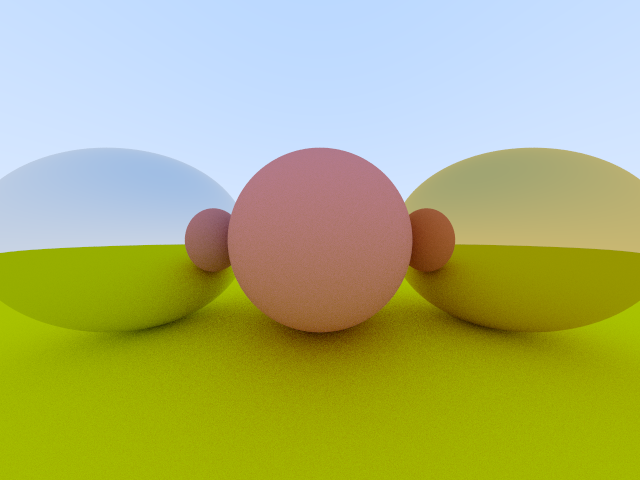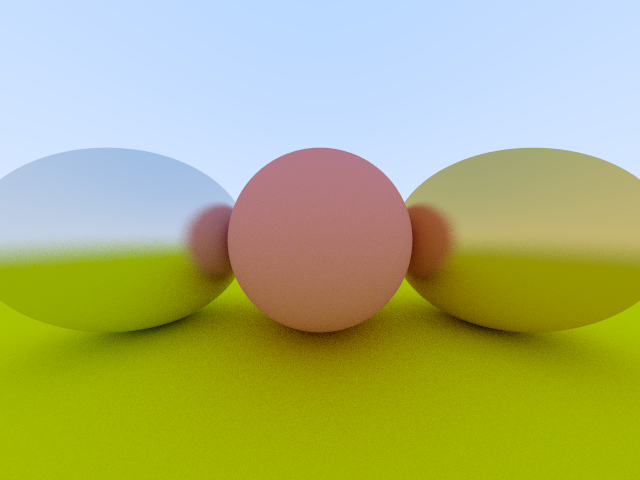]]>
<p>实现简单的金属材质。</p>

## 漫反射材质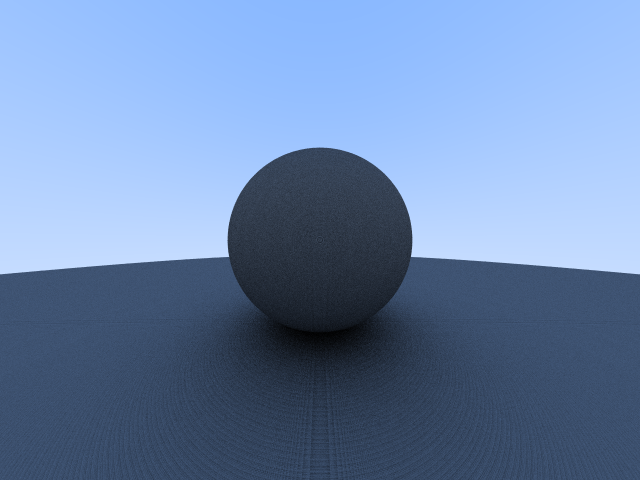## 效果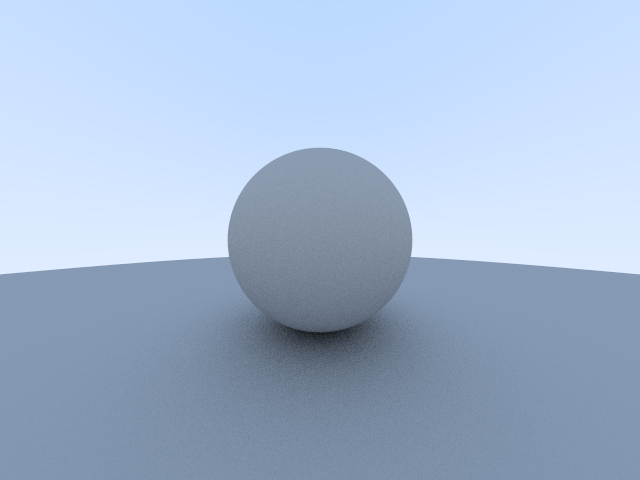]]>
<p>实现简单的漫反射材质。</p>

## 效果]]>
<p>反走样，消除 $5$ 中较为明显的锯齿。</p>

## 法向量## 封装

### Collide

Collide.h

### Sphere

Sphere.h

### CollisibleList

CollisibleList.h

## 效果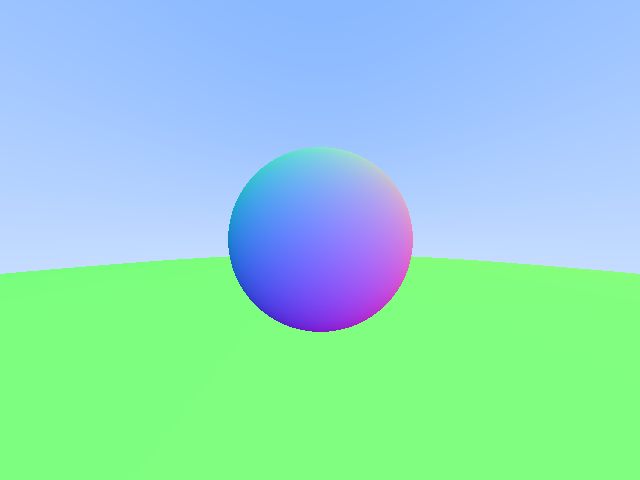]]>
<p>利用球的法向量来着色并简易封装代码实现多个球的渲染。</p>

## 效果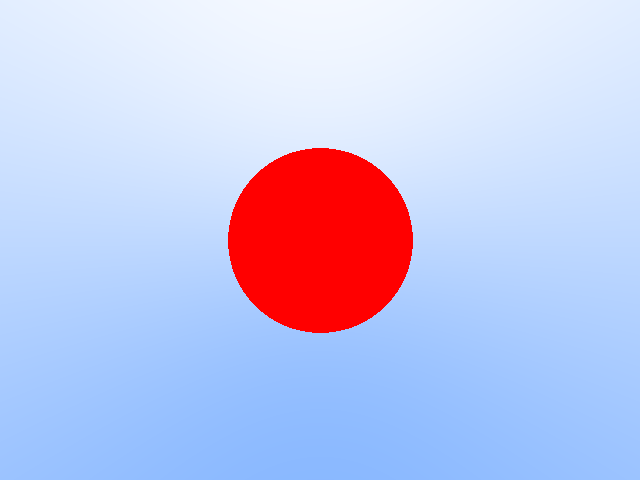]]>
<p>用光线追踪渲染一个球。</p>

### Ray

Ray.h

## 效果

### 按 Y 线性插值### 按 X 线性插值### 按 Z 线性插值]]>
<p>编写光线并测试。</p>

### Vector

]]>
<p>完成 <code>Vector.h</code> 的编写。</p>

### 效果]]>
<p>学习最基础的光线追踪，主要参考 <a href="https://github.com/petershirley/raytracinginoneweekend">Ray Tracing in One Weekend</a>，不同的是我将使用更现代的 C++ 实现，并用 <code>png</code> 格式显示结果。</p>
「LOJ 138」类欧几里得算法 https://blog.xehoth.cc/LOJ138/ 2018-05-18T01:17:57.000Z 2020-02-23T11:20:08.993Z 给出 $T$ 组询问，每组用 $n, a, b, c, k_1, k_2$ 来描述。对于每组询问，请你求出

$$\sum_{x = 0} ^ {n} x ^ {k_1} {\left \lfloor \frac{ax + b}{c} \right \rfloor} ^ {k_2}$$

LOJ 138

### 题解

$$\lfloor \frac{b} {c} \rfloor ^ {k_2} \sum_{x = 0} ^ n x ^ {k_1}$$，此时就是一个自然数幂和，令 $B$ 表示伯努利数，$S_k(n) = \sum\limits_{x = 0} ^ n x ^ k$，则$$S_k(n) = \frac {1} {k + 1}\sum_{j = 0} ^ k (-1) ^ j \binom{k + 1} {j} B_j n ^ {k + 1 - j}$$

\begin{aligned}\sum_{x = 0} ^ n x ^ {k_1} \lfloor \frac {ax + b} {c} \rfloor &= \sum_{x = 1} ^ n x ^ {k_1}(qx + \lfloor \frac{rx + b} {c} \rfloor) ^ {k_2} \\ &= \sum_{i = 0} ^ {k_2} \binom {k_2} {i}q ^ i \sum_{x = 0} ^ n x ^ {k_1 + i}\lfloor \frac{rx + b} {c} \rfloor ^ {k_2 - i}\end{aligned}。

$$\lfloor \frac{cy - b - 1} {a} \rfloor \lt x \leq \lfloor \frac{c(y + 1) - b - 1} {a} \rfloor$$

$$\sum_{x = 0} ^ n x ^ {k_1} \lfloor \frac{ax + b} {c} \rfloor ^ {k_2} = S_{k_1}(n)m ^ {k_2} + \sum_{y = 1} ^ m \big( (y - 1) ^ {k_2} - y ^ {k_2} \big)S_{k_1}(\lfloor \frac{cy - b - 1} {a} \rfloor)$$

$$(y - 1) ^ {k_2} - y ^ {k_2} = \sum_{i = 0} ^ {k_2 - 1}\binom{k_2} {i}(-1) ^ {k_2 - i}y ^ i$$

$$\sum_{x = 0} ^ {n}x ^ {k_1}\lfloor \frac {ax + b} {c} \rfloor ^ {k_2} = S_{k_1}(n)m ^ {k_2} + \sum_{i = 0} ^ {k_2 - 1}\sum_{h = 0} ^ {k_1 + 1}\big(\binom{k_2} {i}(-1) ^ {k_2 - i}P_{k_1, h}\sum_{y = 1} ^ m y ^ i\lfloor \frac{cy - b - 1} {c} \rfloor ^ h \big)$$

### 代码

]]>
<p>给出 $T$ 组询问，每组用 $n, a, b, c, k_1, k_2$ 来描述。对于每组询问，请你求出</p> <p>$$<br>\sum_{x = 0} ^ {n} x ^ {k_1} {\left \lfloor \frac{ax + b}{c} \right \rfloor} ^ {k_2}<br>$$</p> <p>对 $1000000007$ 取模。</p>
「CC MANYLIST」-树状数组 + map https://blog.xehoth.cc/CC-MANYLIST/ 2018-05-12T03:41:56.000Z 2020-02-23T11:20:08.985Z 有 $n$ 个集合，初始都为空，要求支持：

1. 向第 $[l, r]$ 个集合中加入 $x$。
2. 求第 $i$ 个集合的元素个数。

CC MANYLIST

### 代码

]]>
<p>有 $n$ 个集合，初始都为空，要求支持：</p> <ol> <li>向第 $[l, r]$ 个集合中加入 $x$。</li> <li>求第 $i$ 个集合的元素个数。</li> </ol>
「CC GEOCHEAT」-凸包 https://blog.xehoth.cc/CC-GEOCHEAT/ 2018-05-02T07:26:27.000Z 2020-02-23T11:20:08.985Z 每次向平面中添加一个点 $P$，求当前点集的直径（任意两点最大距离）的平方。

CC GEOCHEAT

### 代码

]]>
<p>每次向平面中添加一个点 $P$，求当前点集的直径（任意两点最大距离）的平方。<br><strong>数据随机</strong></p>
「CC PRIMEDST」Prime Distance On Tree-点分治 + FFT https://blog.xehoth.cc/CC-PRIMEDST/ 2018-05-02T04:06:55.000Z 2020-02-23T11:20:08.985Z 给一棵树，随机选取两个点，求两点间路径距离为质数的概率。

CC PRIMEDST

### 题解

$$\sum_{i + j = P}cnt_i \cdot cnt_j$$

### 代码

]]>
<p>给一棵树，随机选取两个点，求两点间路径距离为质数的概率。</p>
「CC SUMDIS」Sum of distances-分治 + 树状数组 https://blog.xehoth.cc/CC-SUMDIS-Sum-of-distances/ 2018-05-02T01:30:43.000Z 2020-02-23T11:20:08.985Z 有一张 $n$ 个节点的有向无环图，节点编号为 $1 \sim n$。图的连边情况如下：

• $\forall 1 \leq i \leq n - 1$，存在一条节点 $i$ 连向节点 $i + 1$ 的边，权值为 $a_i$。
• $\forall 1 \leq i \leq n - 2$，存在一条节点 $i$ 连向节点 $i + 2$ 的边，权值为 $b_i$。
• $\forall 1 \leq i \leq n - 3$，存在一条节点 $i$ 连向节点 $i + 3$ 的边，权值为 $c_i$。

CC SUMDIS

### 题解

1. $f(l, r, m) = \sum\limits_{l \leq i \lt m \lt j \leq r}d(i, j)$
2. $g = \sum\limits_{l \leq i \lt m - 1}d(i, m - 1) + \sum\limits_{l \leq i \lt m}d(i, m) + \sum\limits_{m \lt i \leq r}d(m, i) + \sum\limits_{m + 1 \lt i \leq r}d(m + 1, i)$

$d_1(i, j) = d(i, m - 1) + d(m - 1, j)$
$d_2(i, j) = d(i, m) + d(m, j)$
$d_3(i, j) = d(i, m + 1) + d(m + 1, j)$

$d(m - 1, j) - d(m, j) \leq d(i, m) - d(i, m - 1)$
$d(m - 1, j) - d(m + 1, j) \leq d(i, m + 1) - d(i, m - 1)$

$x_0 = d(i, m) - d(i, m - 1), y_0 = d(i, m + 1) - d(i, m - 1)$
$x_j = d(m - 1, j) - d(m, j), y_j = d(m - 1, j) - d(m + 1, j)$

### 代码

]]>
<p>有一张 $n$ 个节点的<strong>有向无环图</strong>，节点编号为 $1 \sim n$。图的连边情况如下：</p> <ul> <li>$\forall 1 \leq i \leq n - 1$，存在一条节点 $i$ 连向节点 $i + 1$ 的边，权值为 $a_i$。</li> <li>$\forall 1 \leq i \leq n - 2$，存在一条节点 $i$ 连向节点 $i + 2$ 的边，权值为 $b_i$。</li> <li>$\forall 1 \leq i \leq n - 3$，存在一条节点 $i$ 连向节点 $i + 3$ 的边，权值为 $c_i$。</li> </ul> <p>除此之外，图中不存在其它的边。<br>对于一对节点 $s$ 和 $t$ $(s \lt t)$，记 $d(s, t)$ 为从 $s$ 到 $t$ 的最短路径长度。请你求出所有的 $d(s, t)$ 之和，其中 $1 \leq s \lt t \leq n$。</p>